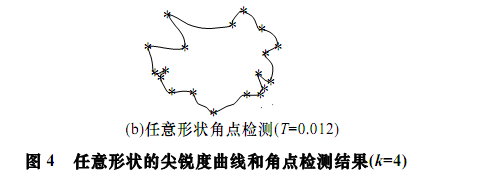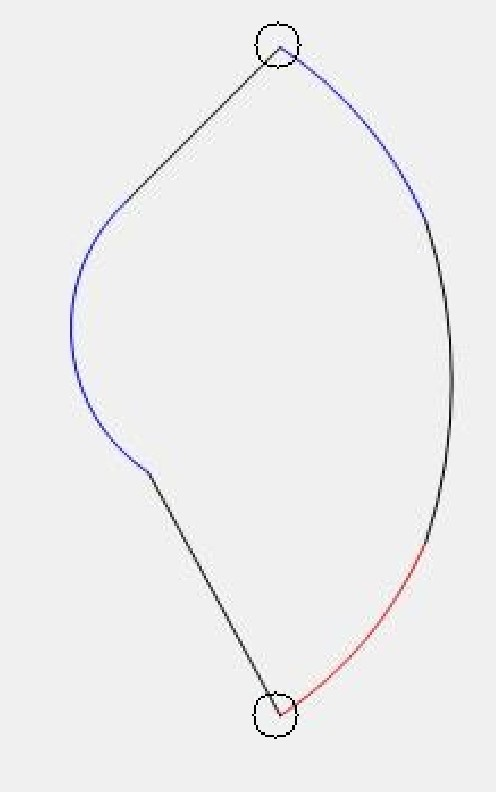# opencv的实用研究--分析轮廓并寻找边界点

opencv的实用研究--分析轮廓并寻找边界点
​      轮廓是图像处理中非常常见的。对现实中的图像进行采样、色彩变化、灰度变化之后，能够处理得到的是“轮廓”。它直接地反应你了需要分析对象的边界特征。而对轮廓的分析，实际上也就是对原图像特征的分析。
在Opencv中，已经实现了基础的轮廓算法，但是相比较于比如halcon这样的专业软件，在轮廓处理这块的功能还是比较缺乏的。这里就通过一个具体问题，说明自己的学习研究。不对之处欢迎批评。
P.S这里的轮廓处理相关函数，已经包涵在GOBase中，具体可以到公告中找Github.

那么如果对于一个简单的图像，比如
已经获得了最大物体的轮廓，比如
//灰度域变化  threshold(gray,gray,0,255,THRESH_BINARY_INV);  GaussianBlur(gray,gray,Size(3,3),0,0);  //寻找和绘制轮廓  VP bigestContour = FindBigestContour(gray);  contours.push_back(bigestContour);

由于在opencv里面，轮廓是以
1. vector<vector<point>>
保存的，那么如何获得这个轮廓的四个顶点了？
尝试直接打印轮廓中第一个点，那么的确是左上角

现在，对于“左上”和“右下”的两个点，是比较好分析的。因为在所有的包含在轮廓中的点中，他们一个是x,y同时最小的，一个是x,y同时最大的。
比较复杂的是“左下”和"右上"两个点，因为他们的数值不是非常有特征，比较容易产生混淆。这个时候，如果仅仅是通过x,y值来分析，即使是对于简单图像，也很难得到稳定的结果。
1. int itopleft =65535;int idownright =0;Point ptopleft;Point pdownright;Point pdownleft;for(int i=0;i<bigestContour.size();i++){//左上if(bigestContour[i].x + bigestContour[i].y <itopleft){itopleft = bigestContour[i].x + bigestContour[i].y ;ptopleft = bigestContour[i];}//右下if(bigestContour[i].x+bigestContour[i].y>idownright){idownright = bigestContour[i].x+bigestContour[i].y;pdownright = bigestContour[i];}}int idownleft =65534;//对于左下的点来说，应该是所有y大于左上的点中，x最小的for(int i=0;i<bigestContour.size();i++){if(bigestContour[i].y>ptopleft.y){if(bigestContour[i].x<idownleft){idownleft = bigestContour[i].x;pdownleft = bigestContour[i];}}}//绘制circle(board,ptopleft,10,Scalar(255),5);circle(board,pdownright,10,Scalar(255),5);circle(board,pdownleft,10,Scalar(255),5);

那么，直观的方法是不稳定的。这个时候，我想到在进行图像处理的时候，有所谓“特征点”的说法。比较常见的比如harris/shift/surf。那么我是否能够通过分析轮廓图像，找到轮廓图像特征点的方法找到我需要的边角了？
编码实现：
///在board上寻找角点///// Detector parameters int blockSize = 2; int apertureSize = 3; double k = 0.04; int thresh = 1;/// Detecting corners board.convertTo(board,CV_32F);cornerHarris( board,dst,2,3,0.04);///// Normalizing normalize( dst, dst_norm, 0, 255, NORM_MINMAX, CV_32FC1, Mat() ); convertScaleAbs( dst_norm, dst_norm_scaled );  ///// Drawing a circle around corners for( int j = 0; j < dst_norm.rows ; j++ )  {        for( int i = 0; i < dst_norm.cols; i++ )  {     if( (int) dst_norm.at<float>(j,i) > thresh )  {  circle( dst_norm_scaled, Point( i, j ), 5,  Scalar(0), 2, 8, 0 );   circle(src,Point( i, j ), 5,  Scalar(255,0,0), -1, 8, 0 ); }  }

NICE，在图像中已经明显的显示出来了4个边界点，再加上已经有的两个点，得到结果不成问题。

但是这里其实是用了一个“投机取巧”的方法，那就是使用图像处理的才使用的harris算法来分析轮廓。opencv默认实现的harris速度慢且会内存移除。用在这个简单的例子里面看似可以，但是无法处理现实问题。所以就必须分析原理。
做图像处理有一段时间了，我经常反思回忆，在图像处理中，能够稳定解决问题的，往往依靠的是“先验知识，本质特征”；越是分析逼近图像的本质特征，越能够发现稳定的解决方法。比如对于轮廓的角来说，很容易想到处于边角的点和两边的点肯定具有一定的关系，而这种关系具有特征性。
所以有目的地寻找论文，很快就有了成果：对于我的研究来说，这篇论文两个贡献：一个是告知首先要对图像进行高斯模糊，这个是我之前没有想到的。特别是对于现实世界中的轮廓，这种方法效果很好。因为边角经过模糊，那么还是边角，但毛刺经过模糊，能够有效去除。
论文中的算法实现是比较简单的，并且给出了简化算法，直接编码验证：
1.  //遍历轮廓，求出所有支撑角度    int icount = bigestContour.size();    float fmax = -1;//用于保存局部最大值    int   imax = -1;    bool  bstart = false;    for (int i=0;i<bigestContour.size();i++){        Point2f pa = (Point2f)bigestContour[(i+icount-7)%icount];        Point2f pb = (Point2f)bigestContour[(i+icount+7)%icount];        Point2f pc = (Point2f)bigestContour[i];        //两支撑点距离        float fa = getDistance(pa,pb);        float fb = getDistance(pa,pc)+getDistance(pb,pc);        float fang = fa/fb;        float fsharp = 1-fang;        if (fsharp>0.05){            bstart = true;            if (fsharp>fmax){                fmax = fsharp;                imax = i;            }        }else{            if (bstart){                circle(board,bigestContour[imax],10,Scalar(255),1);                circle(src,bigestContour[imax],10,Scalar(255,255,255),1);                imax  = -1;                fmax  = -1;                bstart = false;            }        }    }

编码过程中，相比较于原文，有两处优化（原文中应该也提到了，但是没有明说）：一是通过取模，使得所有的轮廓点都参与运算；二是通过比较，取出角点的局部最大值。
实现效果，比较理想：

1、掌握知识，如果不能归纳数学模型，并且编码实现，不叫真正掌握；
2、分析研究，如果从简单的情况开始，控制变量，往往能够左右逢源。

P.S 网友提问
好，测试的图片是这样的，需要把图片里的2段圆弧与2条直线分割出来，《基于轮廓尖锐度的图像角点检测算法》所实现的算法，不清楚能不能实现#include <opencv2\highgui.hpp>
#include <opencv2\opencv.hpp>
#include "GOCVHelper.h"
#include <iostream>
using namespace std;
using namespace cv;
int main() {
Mat src = imread("e:/sandbox/测试图片.jpg"IMREAD_COLOR);
Mat gray;
Mat board(src.size()src.type(), Scalar::all(0));
vector<VPcontours;
//灰度域变化
cvtColor(srcgrayCOLOR_BGR2GRAY);
threshold(graygray, 100, 255, THRESH_OTSU);
bitwise_not(graygray);
GaussianBlur(graygraySize(3, 3), 0, 0);
//寻找和绘制轮廓
VP bigestContour = FindBigestContour(gray);
contours.push_back(bigestContour);
//遍历轮廓，求出所有支撑角度
int icount = bigestContour.size();
float fmax = -1;//用于保存局部最大值
int   imax = -1;
bool  bstart = false;
for (int i = 0; i < bigestContour.size(); i++) {
Point2f pa = (Point2f)bigestContour[(i + icount - 7) % icount];
Point2f pb = (Point2f)bigestContour[(i + icount + 7) % icount];
Point2f pc = (Point2f)bigestContour[i];
//两支撑点距离
float fa = getDistance(papb);
float fb = getDistance(papc) + getDistance(pbpc);
float fang = fa / fb;
float fsharp = 1 - fang;
if (fsharp > 0.05) {
bstart = true;
if (fsharp > fmax) {
fmax = fsharp;
imax = i;
}
}
else {
if (bstart) {
circle(boardbigestContour[imax], 10, Scalar(255), 1);
circle(srcbigestContour[imax], 10, Scalar(0, 0, 0), 1);
imax = -1;
fmax = -1;
bstart = false;
}
}
}
imshow("src"src);
waitKey(0);
}

posted @ 2020-07-20 13:51  jsxyhelu  阅读(423)  评论(0编辑  收藏  举报